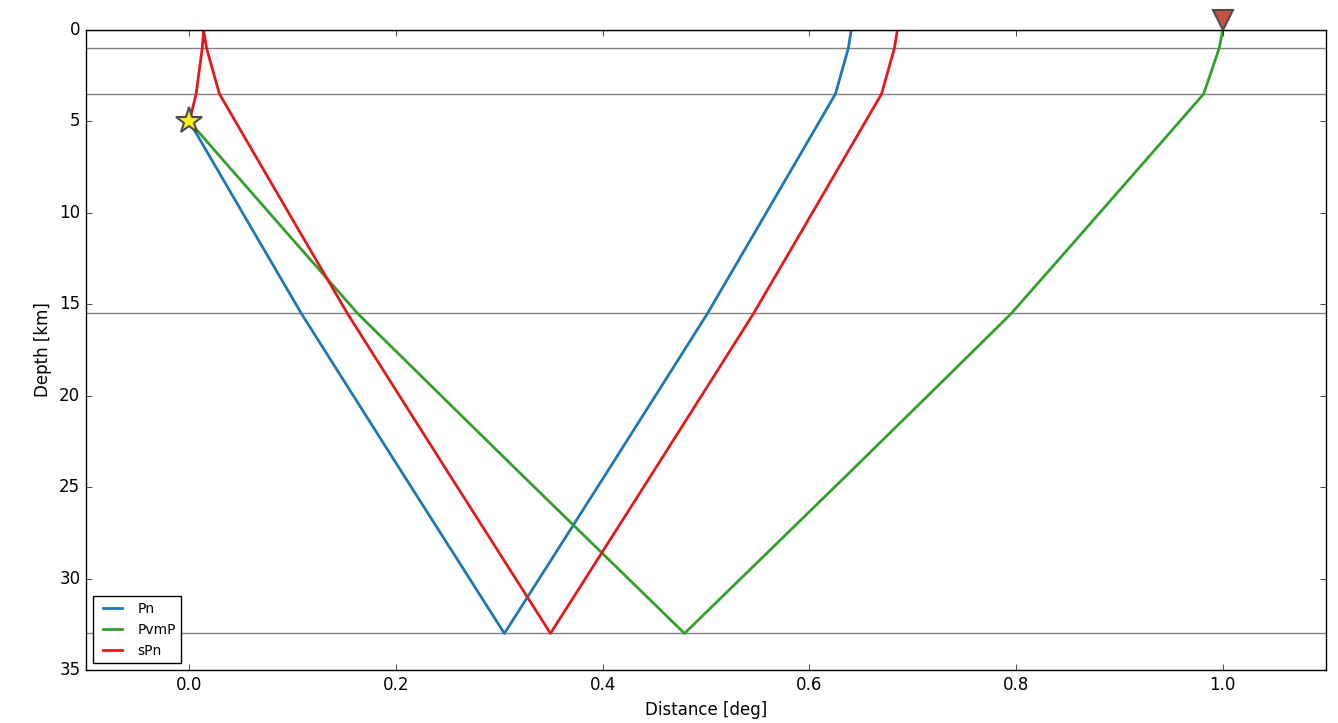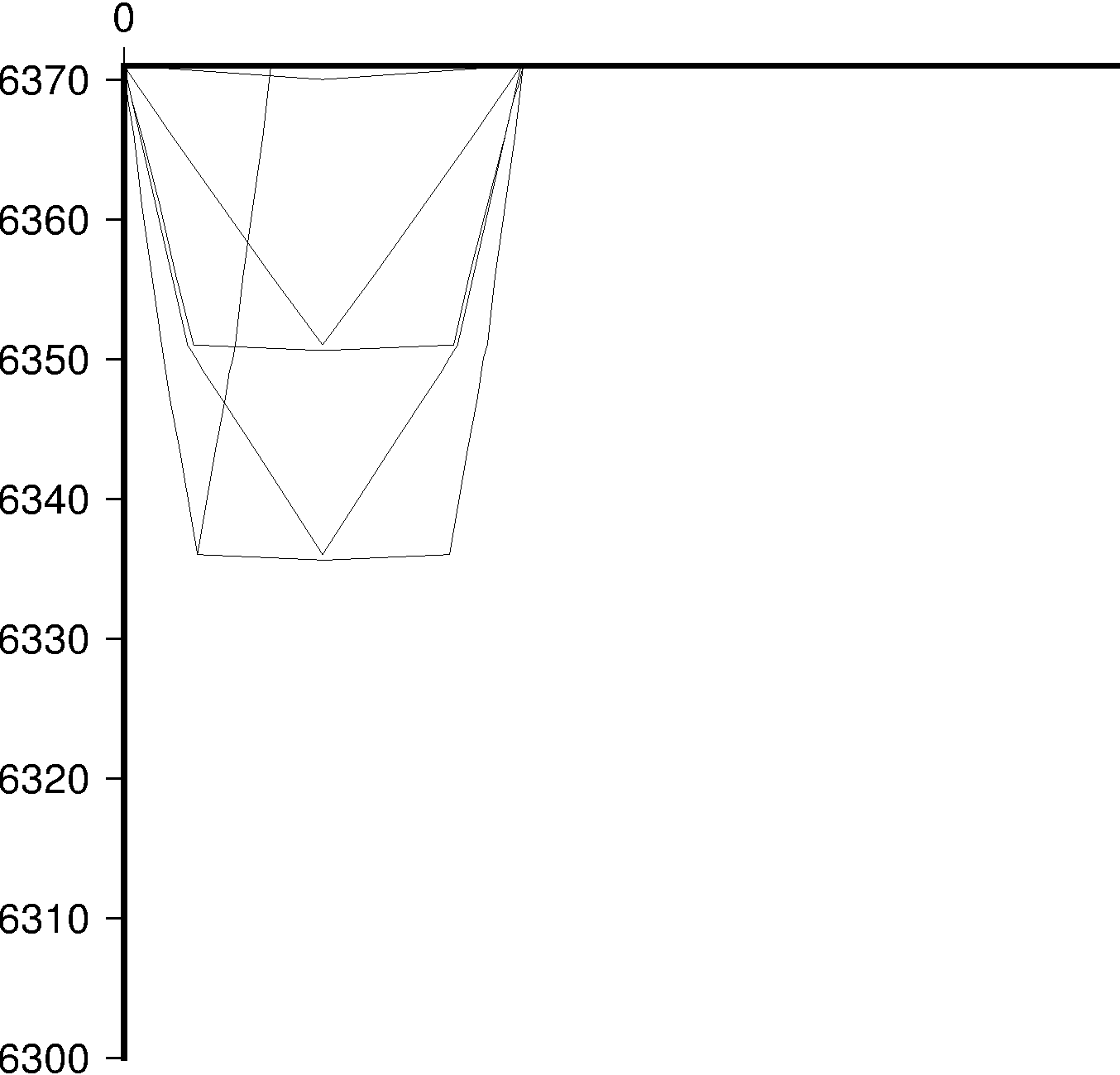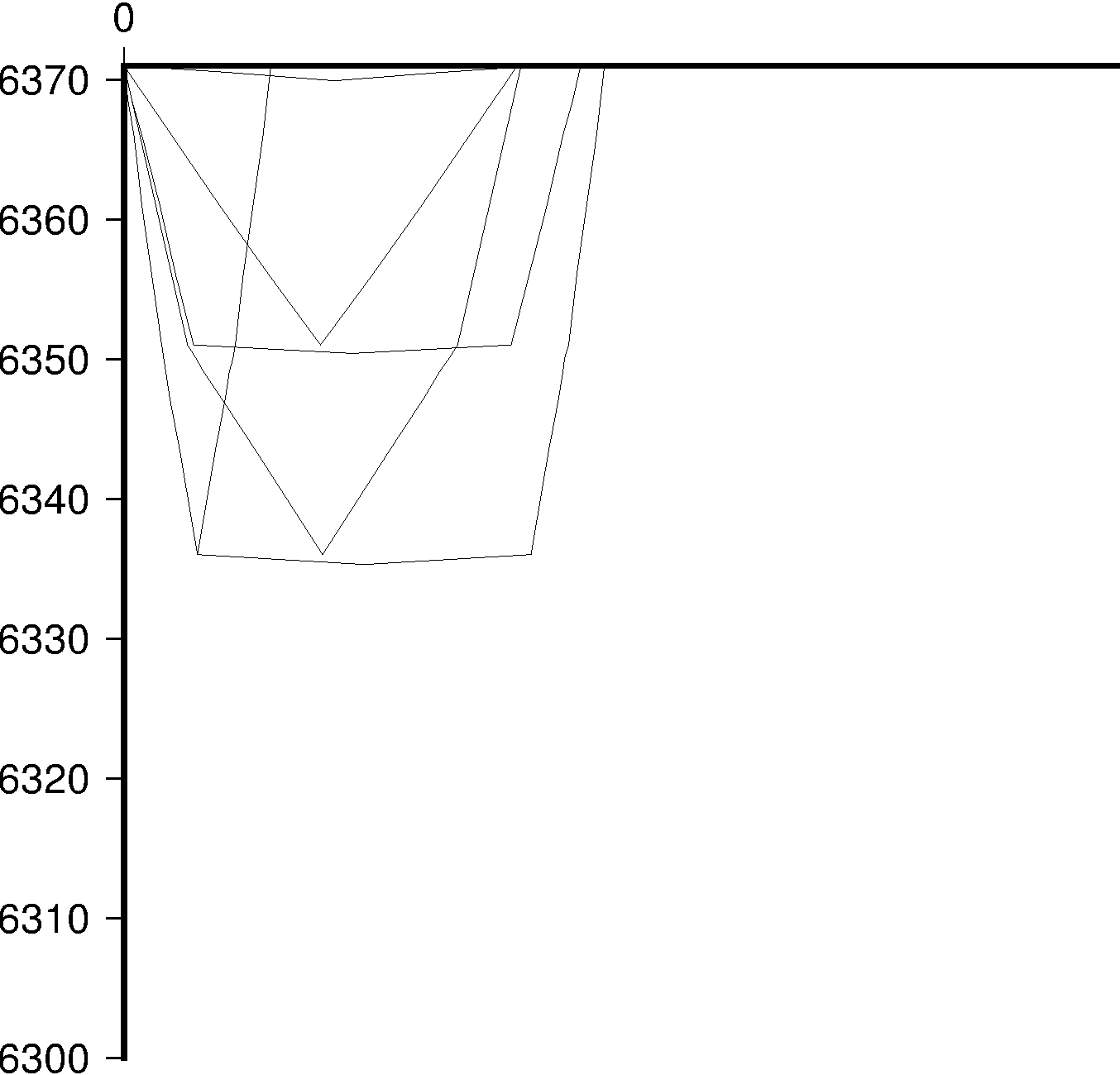# Taup procedure prolem

Hi all

from obspy.taup import TauPyModel
model = TauPyModel(model=“qguo”)
arrivals = model.get_travel_times(source_depth_in_km=5,distance_in_degree=1.278)
print(arrivals)
arrivals = model.get_ray_paths(source_depth_in_km=5,distance_in_degree=1,phase_list=[“Pn”,“sPn”,“PvmP”])
arrivals.plot(plot_type=“cartesian”)

The above codes is used to make the following plot.

Is this have a problem in the phase sPn, Pn or PvmP? the sPn and Pn do not get to the station(triangle), The angle of incidence of Pn is smaller than PvmP, is it right?Junqing

Hi Junging,

the issue is likely similar to this here:
https://github.com/obspy/obspy/issues/1392

Can you work with us on github to resolve this?

Cheers!

Lion

which version of TauP is loaded in Obspy by importing TauPyModel?
I'm currently using the following version
updated to 17.12.2015.
TauP-2.3.0-SNAPSHOT.tgz

maybe in this version the issue is solved.

Luigia

Hi Luigia,

we don't load TauP but ported it. It was originally based on version
2.2. I think but should be pretty much up-to-date with the latest snapshot.

Does the mentioned problem exist in the Java version - if not then it
should be fairly simple to figure out what is wrong with ours.

Cheers!

Lion

Yes,
my email was to point that the problem seems to me to be in the Java version.
I attach a quick plot I made to show the differences between the 2 before mentioned versions of TauP program in the calculation of the raypaths.
In the newer version the rays are reaching the receiver position(sorry no triangle is placed in the receiver location).

Luigia